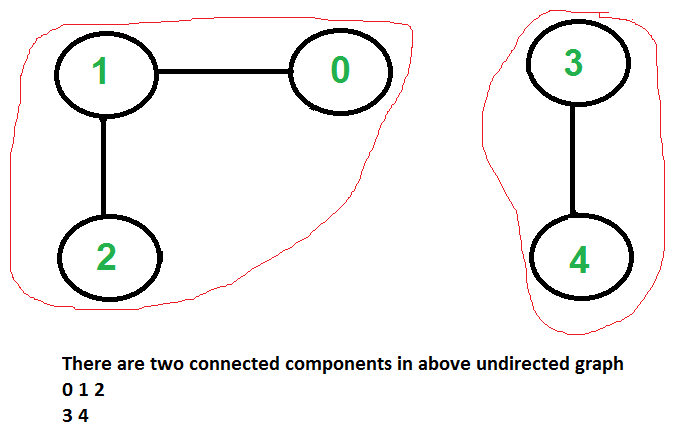Connected Components in an undirected graph

• Difficulty Level : Medium
• Last Updated : 06 Oct, 2021

Given an undirected graph, print all connected components line by line. For example consider the following graph.We strongly recommend to minimize your browser and try this yourself first.
We have discussed algorithms for finding strongly connected components in directed graphs in following posts.
Kosaraju’s algorithm for strongly connected components
Tarjan’s Algorithm to find Strongly Connected Components
Finding connected components for an undirected graph is an easier task. We simple need to do either BFS or DFS starting from every unvisited vertex, and we get all strongly connected components. Below are steps based on DFS.

1) Initialize all vertices as not visited.
2) Do following for every vertex 'v'.
(a) If 'v' is not visited before, call DFSUtil(v)
(b) Print new line character

DFSUtil(v)
1) Mark 'v' as visited.
2) Print 'v'
3) Do following for every adjacent 'u' of 'v'.
If 'u' is not visited, then recursively call DFSUtil(u)

Below is the implementation of above algorithm.

Python

 # Python program to print connected# components in an undirected graph  class Graph:     # init function to declare class variables    def __init__(self, V):        self.V = V        self.adj = [[] for i in range(V)]     def DFSUtil(self, temp, v, visited):         # Mark the current vertex as visited        visited[v] = True         # Store the vertex to list        temp.append(v)         # Repeat for all vertices adjacent        # to this vertex v        for i in self.adj[v]:            if visited[i] == False:                 # Update the list                temp = self.DFSUtil(temp, i, visited)        return temp     # method to add an undirected edge    def addEdge(self, v, w):        self.adj[v].append(w)        self.adj[w].append(v)     # Method to retrieve connected components    # in an undirected graph    def connectedComponents(self):        visited = []        cc = []        for i in range(self.V):            visited.append(False)        for v in range(self.V):            if visited[v] == False:                temp = []                cc.append(self.DFSUtil(temp, v, visited))        return cc  # Driver Codeif __name__ == "__main__":     # Create a graph given in the above diagram    # 5 vertices numbered from 0 to 4    g = Graph(5)    g.addEdge(1, 0)    g.addEdge(2, 3)    g.addEdge(3, 4)    cc = g.connectedComponents()    print("Following are connected components")    print(cc) # This code is contributed by Abhishek Valsan

Javascript



Output:

0 1
2 3 4

Time complexity of above solution is O(V + E) as it does simple DFS for given graph.

This can be solved using Disjoint Set Union with a time complexity of O(N). The DSU solution is easier to understand an implement.

Algorithm :

!. Declare an array arr of size n where n is the total number of nodes.

2. For every index i of array arr, the value denotes who the parent of i’th vertex is. For example is arr=3, then we can say that the parent of vertex 0 is 3.

3. Initialise every node as the parent of itself and then while adding them together, change their parents accordingly.

C++

 #include using namespace std;int merge(int* parent, int x){    if (parent[x] == x)        return x;    return merge(parent, parent[x]);}int connectedcomponents(int n, vector >& edges){    int parent[n];    for (int i = 0; i < n; i++) {        parent[i] = i;    }    for (auto x : edges) {        parent[merge(parent, x)] = merge(parent, x);    }    int ans = 0;    for (int i = 0; i < n; i++) {        ans += (parent[i] == i);    }    for (int i = 0; i < n; i++) {        parent[i] = merge(parent, parent[i]);    }    map > m;    for (int i = 0; i < n; i++) {        m[parent[i]].push_back(i);    }    for (auto it = m.begin(); it != m.end(); it++) {        list l = it->second;        for (auto x : l) {            cout << x << " ";        }        cout << endl;    }    return ans;}int main(){    int n = 5;    vector e1 = { 0, 1 };    vector e2 = { 2, 3 };    vector e3 = { 3, 4 };    vector > e;    e.push_back(e1);    e.push_back(e2);    e.push_back(e3);    int a = connectedcomponents(n, e);    cout << "total no. of connected components are: " << a         << endl;    return 0;}
Output
0 1
2 3 4
total no. of connected components are: 2